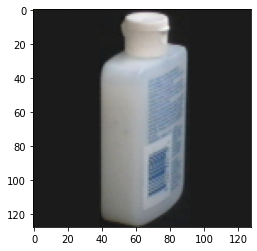Learn data science and machine learning by building real-world projects on Jovian

#Image classification with the Coil-100 dataset

COIL-100 was collected by the Center for Research on Intelligent Systems at the Department of Computer Science , Columbia University. The database contains color images of 100 objects. The objects were placed on a motorized turntable against a black background and images were taken at pose internals of 5 degrees. This dataset was used in a real-time 100 object recognition system whereby a system sensor could identify the object and display its angular pose.

In this example, I will utilize transfer learning with the VGG-11 pre-trained convolutional network. I will add a custom classification layer to it and train it on GPU.

In [ ]:
``````import opendatasets as od
dataset_url = 'https://www.kaggle.com/jessicali9530/coil100'
``````
```Please provide your Kaggle credentials to download this dataset. Learn more: http://bit.ly/kaggle-creds Your Kaggle username: triaprima Your Kaggle Key: ·········· ```
` 21%|██▏ | 27.0M/127M [00:00<00:00, 276MB/s]`
```Downloading coil100.zip to ./coil100 ```
```100%|██████████| 127M/127M [00:00<00:00, 297MB/s] ```
``` ```
In [ ]:
``````import os, glob
import torch
import pandas as pd
import numpy as np
from torch.utils.data import Dataset, random_split, DataLoader
from PIL import Image, ImageStat
import torchvision.models as models
from torch import optim
import matplotlib.pyplot as plt
import torchvision.transforms as transforms
from torchvision.utils import make_grid
import torch.nn.functional as F
import torch.nn as nn
from torchvision.utils import make_grid
from collections import OrderedDict
%matplotlib inline
``````
In [ ]:
``````DATA_DIR = './coil100/coil-100/coil-100'
file_list = glob.glob(f'{DATA_DIR}/*.png')
# extracting file names and related labels
f_names_labels = [(f.split('/')[-1], int(f.split('/')[-1].split('__').split('obj')))   for f in file_list]``````

Note: the labels of the objects go from 1 to 100, while the output tensor of the neural network will have indexes that go from 0 to 99, and this will be taken into account below.

In [ ]:
``````class Coil100(Dataset):
def __init__(self, f_names_labels_list, root_dir, transform=None):
self.df = f_names_labels_list
self.transform = transform
self.root_dir = root_dir

def __len__(self):
return len(self.df)

def __getitem__(self, idx):
row = self.df[idx]
img_id, img_label = row, row - 1 # image file name and label (label number will start from 0 instead of 1)
img_fname = self.root_dir + "/" + str(img_id)
img = Image.open(img_fname)
if self.transform:
img = self.transform(img)
return img, img_label``````

I choose not to normalized the images, unless the results obtained are not satisfying.

In :
``````transform = transforms.Compose([transforms.ToTensor()])
dataset = Coil100(f_names_labels, DATA_DIR, transform=transform)
len(dataset)``````
Out:
``7200``

Getting a sample:

In :
``````def show_sample(img, target, invert=True):
if invert:
plt.imshow(1 - img.permute((1, 2, 0)))
else:
plt.imshow(img.permute(1, 2, 0))
print('Label:', target)``````
In :
``show_sample(*dataset, invert=False)``
```Label: 12 ```Splitting the dataset into a training, validation and test datasets.

In [ ]:
``````dlen = len(dataset)

train_size, test_size, validation_size = int(0.8 * dlen), int(0.1 * dlen), int(0.1 * dlen)

train_dataset, test_dataset, validation_dataset = torch.utils.data.random_split(dataset, [train_size, test_size, validation_size])

print([len(f) for f in (train_dataset, test_dataset, validation_dataset)])``````
```[5760, 720, 720] ```

Initializing dataloader per each of them.

In [ ]:
``````batch_size = 64

train_dl = DataLoader(train_dataset, batch_size, shuffle=True, num_workers=4, pin_memory=True)
valid_dl = DataLoader(validation_dataset, batch_size*2, num_workers=4, pin_memory=True)
test_dl = DataLoader(test_dataset, batch_size*2, num_workers=4, pin_memory=True)``````
In :
``````def show_batch(dl):
for images, labels in dl:
fig, ax = plt.subplots(figsize=(16, 16))
ax.set_xticks([]); ax.set_yticks([])
ax.imshow(make_grid(images.cpu(), nrow=8).permute(1, 2, 0))
break``````

Picking a random batch: the same object can appear in multiple angles in the same batch.

In :
``show_batch(train_dl)``